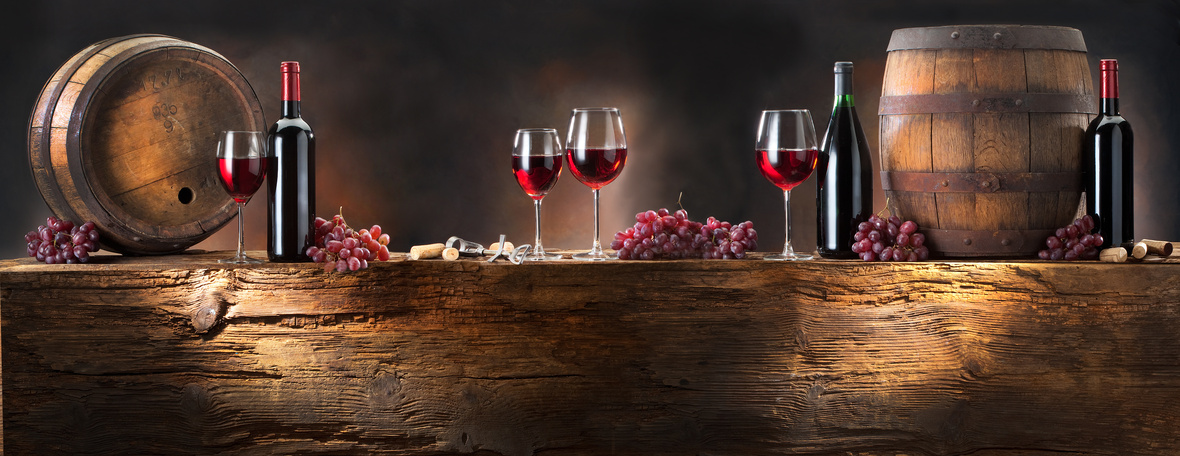Autumn Libations, Rewards for Wine Lovers and BIG NEWS!

 /* styles */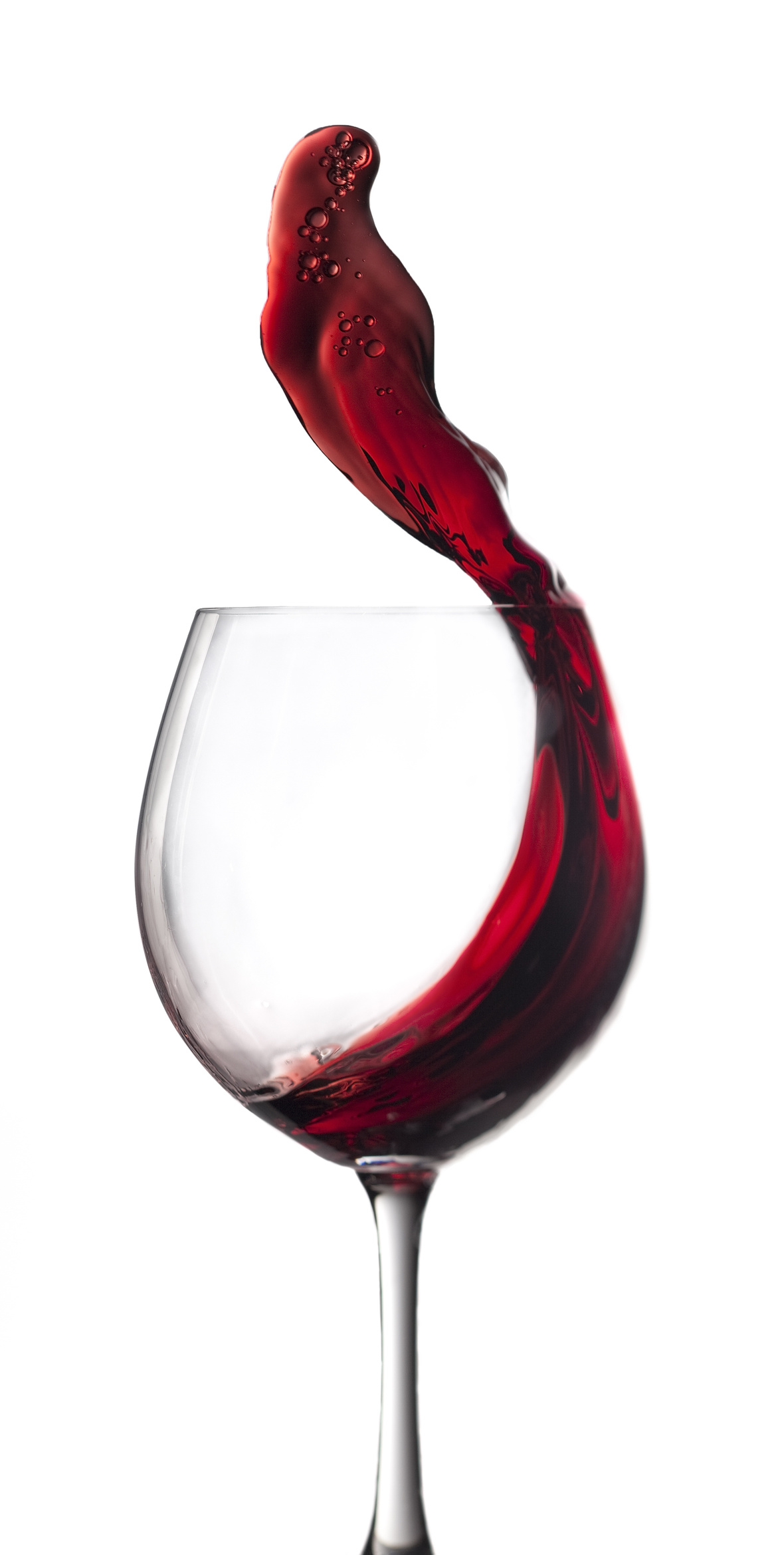Hi Wine & Spirits Lover,

The Shall We Wine team is excited to share our monthly newsletter. Our goal is to introduce you to our favorite selections, producers, distillers, varietals, regions and places to purchase and drink fabulous wine and spirits!

Cheers,

The Shall We Wine Team

 /* styles */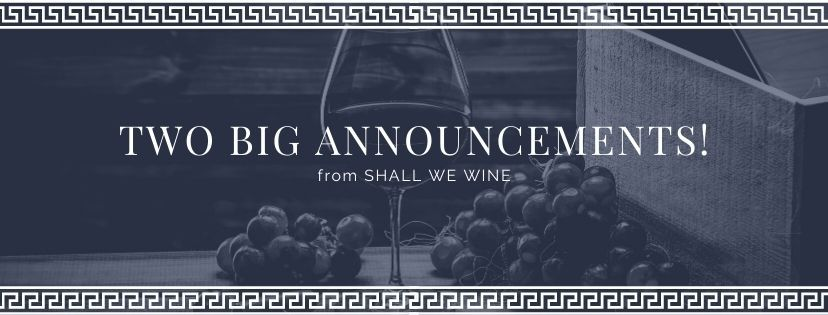table div table+table+table+table+table+table+table div table{width:100%;padding:0}table div table+table+table+table+table+table+table div table img{width:96.23%;padding:0;float:none}table div table+table+table+table+table+table+table div table td{width:100%;padding:0 1.88% 18px}/* styles */BIG NEWS! THE SWW MEMBERSHIPThe Ultimate Sip of Approval

What started as a gathering of wine and spirits lovers formed a community where members come to share, learn, and grow.

Our members are people who are interested in exploring wine, spirits, travel, books, art, health, wellness, advocacy and having deep and fun conversation around these interests.

After months of hard work, we are inviting you to take a seat at our table.

 /* styles */ Shall We Wine Membership offers curated wine and spirits events led by industry. We offer a safe space to have thought-provoking conversations, and when the world allows us, we will take our members to our favorite destinations! PEEK IN View our new website and check out past events on the SWW YouTube Channel! TAKE A SEAT Experience our membership through our virtual Cocktail Hour THIS FRIDAY, October 16th! JOIN OUR COMMUNITY Choose a membership from our four options: Village, Cru, Premier Cru and Grand Cru. You are the guest of honor. Shall We Wine Membership was developed based on feedback from our current followers. As Brenee Brown says, we are here to “get it right, not be right.” We are proud of what we have put together but are open to adding new components based on the needs of our community.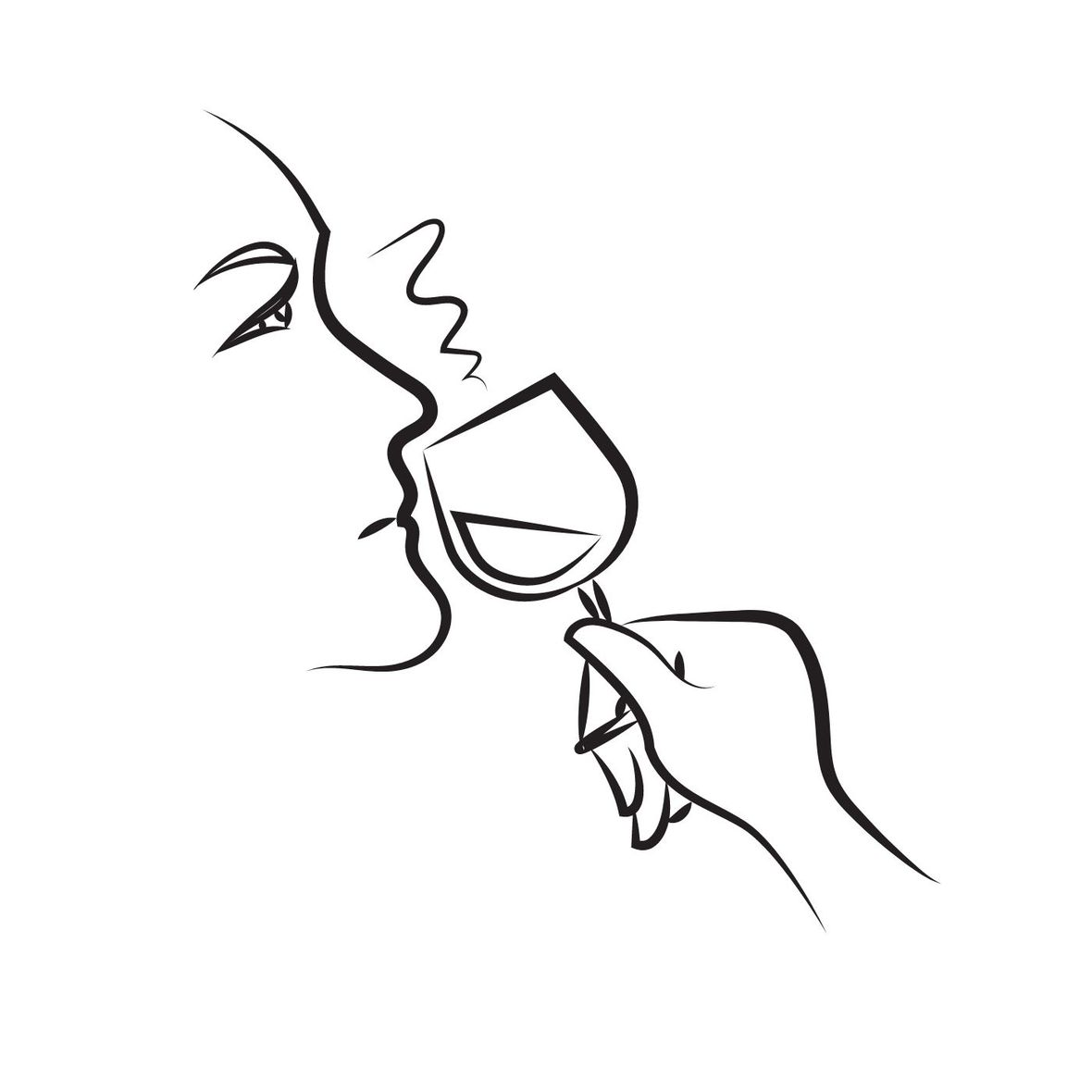[*] Our followers love our virtual Cocktail Hour events. As a Cru member, you will have access to two to three events per month.

[*] Our followers asked for more educational programming. As a Premier Cru member you will have access to a library of Fundamentals wine and spirits classes.

[*] Our followers asked for an ultimate VIP experience. Schedule a call with me for details and an application to our Grand Cru Membership.

Membership to Shall We Wine is our way of recognizing our most loyal wine tribe ⁠— the best way to access and take advantage of members-only perks.

This is just the beginning. We have so much more in store for you! Feel free to send me some feedback!Free Wine Book:

Register for our October 16 virtual Cocktail Hour event and receive a free download when you attend!

 table div table+table+table+table+table+table+table+table+table+table+table+table+table div table{width:100%;padding:0}table div table+table+table+table+table+table+table+table+table+table+table+table+table div table img{width:96.23%;padding:0;float:none}table div table+table+table+table+table+table+table+table+table+table+table+table+table div table td{width:100%;padding:0 1.88% 18px}/* styles */BIG NEWS! WINE ENTHUSIAST NOMINATION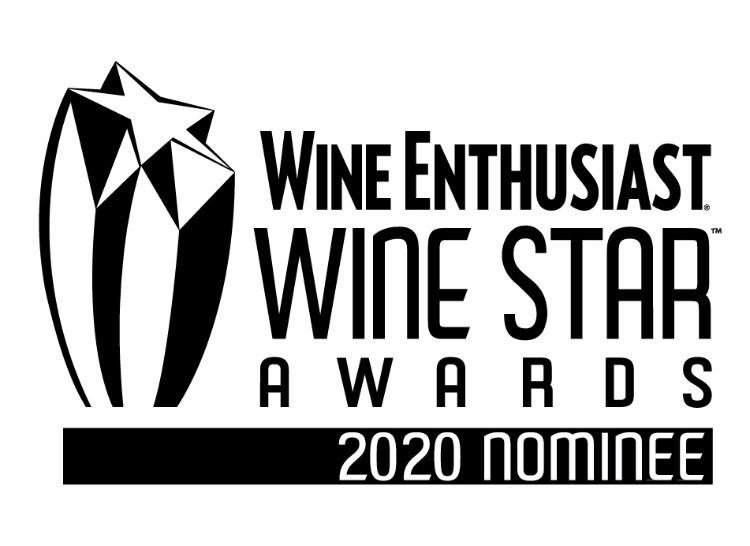We are overjoyed to announce that our Founder Regine T. Rousseau has been nominated for Wine Enthusiast’s 21st Annual Wine Star Award as 2020's Wine Educator of the Year! There are nominees across 15 categories for this year's Wine Star Awards. What an honor to be nominated alongside other incredible movers, shakers and disruptors in the wine world. Congratulations to all nominees!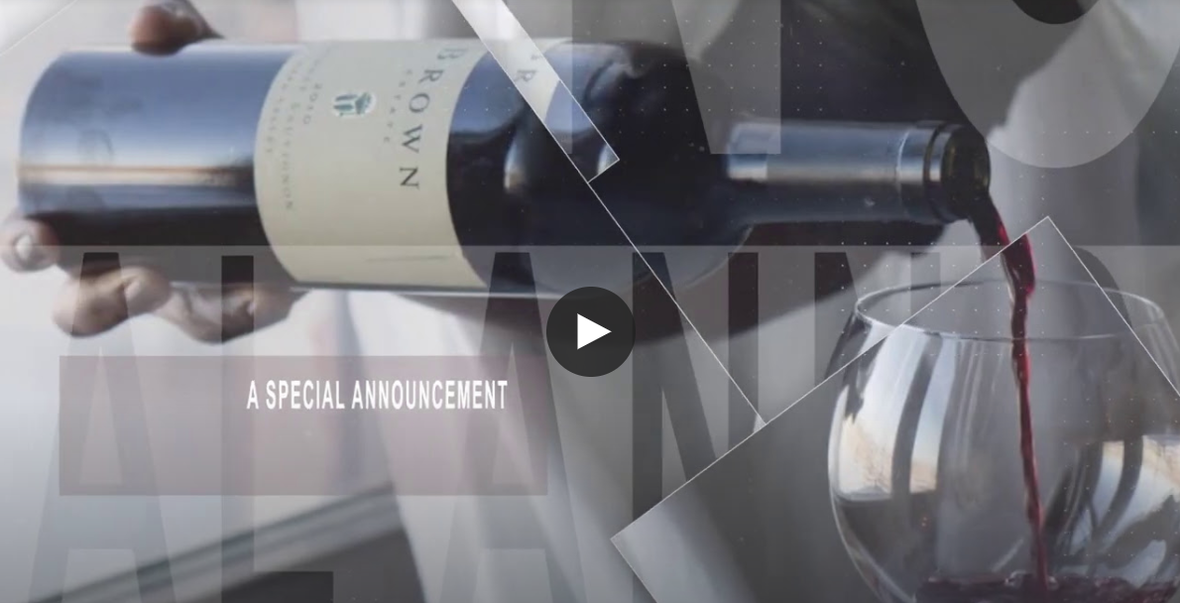NOMINEE ANNOUNCEMENT - CLICK IMAGE TO WATCH VIDEO
 table div table+table+table+table+table+table+table+table+table+table+table+table+table+table+table+table+table div table{width:100%;padding:0}table div table+table+table+table+table+table+table+table+table+table+table+table+table+table+table+table+table div table img{width:96.23%;padding:0;float:none}table div table+table+table+table+table+table+table+table+table+table+table+table+table+table+table+table+table div table td{width:100%;padding:0 1.88% 18px}/* styles */CHECK THIS OUT!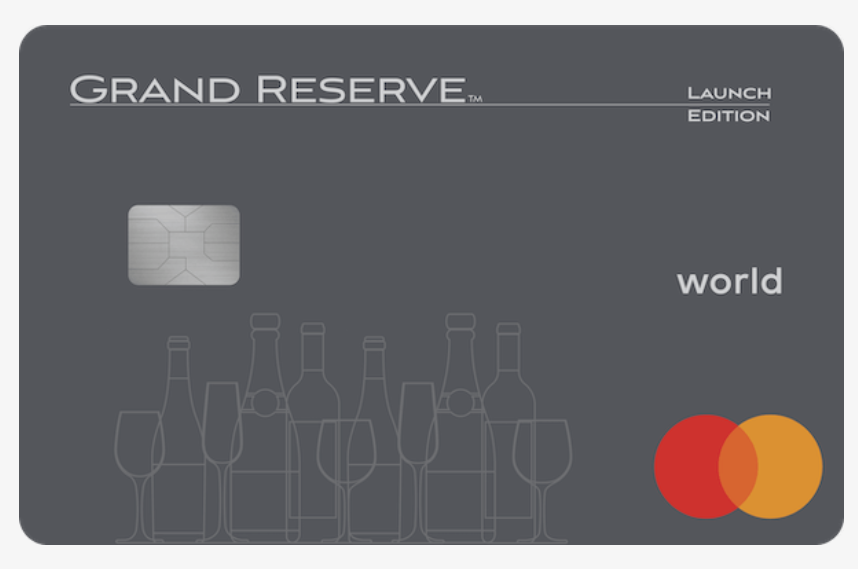Grand Reserve Credit Card - Rewards for Wine Lovers!

PASADENA, Calif., October 7, 2020 – Vertical Finance today announced that they have welcomed 28 new partner wineries to the Grand Reserve Rewards network, a unique loyalty and rewards program centered on the Grand Reserve™ World Mastercard®, the credit card designed for wine enthusiasts.

Full details about Grand Reserve and the Grand Reserve World Mastercard be found at grandreserverewards.com.

Disclaimer: Shall We Wine is not affiliated with Grand Reserve Credit Card or its partners. We are simply sharing news that interest wine lovers.

 table div table+table+table+table+table+table+table+table+table+table+table+table+table+table+table+table+table+table+table+table div table{width:100%;padding:0}table div table+table+table+table+table+table+table+table+table+table+table+table+table+table+table+table+table+table+table+table div table img{width:96.23%;padding:0;float:none}table div table+table+table+table+table+table+table+table+table+table+table+table+table+table+table+table+table+table+table+table div table td{width:100%;padding:0 1.88% 18px}/* styles */NEVER MISS A WINE & SPIRITS CONNECTION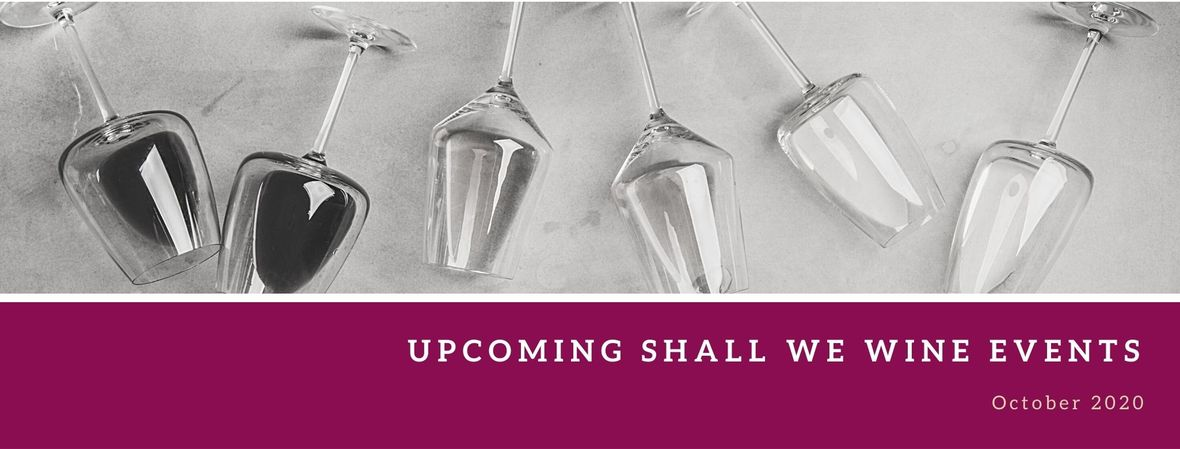/* styles */ Check out our newly updated events calendar to see what your next pour should be!table div table+table+table+table+table+table+table+table+table+table+table+table+table+table+table+table+table+table+table+table+table+table+table+table+table+table div table{width:100%;padding:0}table div table+table+table+table+table+table+table+table+table+table+table+table+table+table+table+table+table+table+table+table+table+table+table+table+table+table div table img{width:96.23%;padding:0;float:none}table div table+table+table+table+table+table+table+table+table+table+table+table+table+table+table+table+table+table+table+table+table+table+table+table+table+table div table td{width:100%;padding:0 1.88% 18px}/* styles */YOUTUBE MANIA: SHALL WE WINE TV

 /* styles */ Did you know SWW had a YouTube Channel? Cool tips, tricks and insider scoop. Check it out! →
 table div table+table+table+table+table+table+table+table+table+table+table+table+table+table+table+table+table+table+table+table+table+table+table+table+table+table+table+table+table div table td,table.module-28{width:100%;padding:0}table div table+table+table+table+table+table+table+table+table+table+table+table+table+table+table+table+table+table+table+table+table+table+table+table+table+table+table+table+table div table{width:100%;float:none;margin-left:auto;margin-right:auto;padding:0}table div table+table+table+table+table+table+table+table+table+table+table+table+table+table+table+table+table+table+table+table+table+table+table+table+table+table+table+table+table div table a{border:0 none;text-decoration:none}table div table+table+table+table+table+table+table+table+table+table+table+table+table+table+table+table+table+table+table+table+table+table+table+table+table+table+table+table+table div table img{width:100%!important;border:0 none;text-decoration:none}/* styles */
 /* styles */
 /* styles */ To stay in the know with the latest and greatest. make sure to follow us all over social!/* styles */ CLICK BELOW
 table div table+table+table+table+table+table+table+table+table+table+table+table+table+table+table+table+table+table+table+table+table+table+table+table+table+table+table+table+table+table+table+table+table+table div table{width:100%;padding:0}table div table+table+table+table+table+table+table+table+table+table+table+table+table+table+table+table+table+table+table+table+table+table+table+table+table+table+table+table+table+table+table+table+table+table div table table{padding:0;float:left!important;width:26.059%!important}table div table+table+table+table+table+table+table+table+table+table+table+table+table+table+table+table+table+table+table+table+table+table+table+table+table+table+table+table+table+table+table+table+table+table div table td{padding-left:29px;padding-right:29px}table div table+table+table+table+table+table+table+table+table+table+table+table+table+table+table+table+table+table+table+table+table+table+table+table+table+table+table+table+table+table+table+table+table+table div table table+table,table div table+table+table+table+table+table+table+table+table+table+table+table+table+table+table+table+table+table+table+table+table+table+table+table+table+table+table+table+table+table+table+table+table+table div table table+table+table{float:left!important;width:26.059%!important}table div table+table+table+table+table+table+table+table+table+table+table+table+table+table+table+table+table+table+table+table+table+table+table+table+table+table+table+table+table+table+table+table+table+table div table table td,table div table+table+table+table+table+table+table+table+table+table+table+table+table+table+table+table+table+table+table+table+table+table+table+table+table+table+table+table+table+table+table+table+table+table div table table+table td,table div table+table+table+table+table+table+table+table+table+table+table+table+table+table+table+table+table+table+table+table+table+table+table+table+table+table+table+table+table+table+table+table+table+table div table table+table+table td{padding-left:0;padding-right:20px}table div table+table+table+table+table+table+table+table+table+table+table+table+table+table+table+table+table+table+table+table+table+table+table+table+table+table+table+table+table+table+table+table+table+table div table table+table+table+table{float:left!important;width:21.822999999999993%!important}table div table+table+table+table+table+table+table+table+table+table+table+table+table+table+table+table+table+table+table+table+table+table+table+table+table+table+table+table+table+table+table+table+table+table div table table+table+table+table td{padding-left:0;padding-right:0}/* styles */
 table div table+table+table+table+table+table+table+table+table+table+table+table+table+table+table+table+table+table+table+table+table+table+table+table+table+table+table+table+table+table+table+table+table+table+table div table{width:100%;padding:0}table div table+table+table+table+table+table+table+table+table+table+table+table+table+table+table+table+table+table+table+table+table+table+table+table+table+table+table+table+table+table+table+table+table+table+table div table img{width:96.23%;padding:0;float:none}table div table+table+table+table+table+table+table+table+table+table+table+table+table+table+table+table+table+table+table+table+table+table+table+table+table+table+table+table+table+table+table+table+table+table+table div table td{width:100%;padding:0 1.88% 18px}/* styles */DISCOUNT OFFER FOR YOU: ARTWORK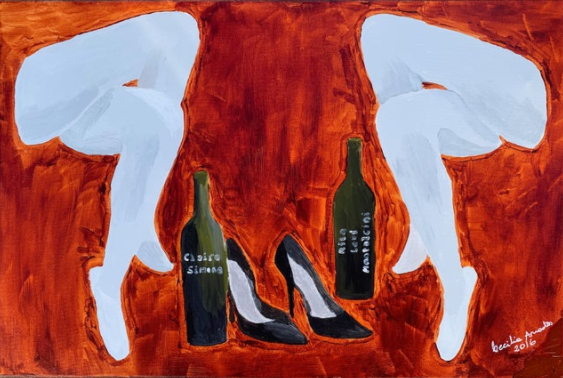Who doesn't love a discount?!

Shall We Wine is putting you on to the amazing Cecilia Anastos Paintings. In addition, there is a discount offer available to the Shall We Wine tribe!

Coupon Code: SWW2020
Expires: Oct 31st, 2020

Free shipping in the USA and local pick-up in San Diego, CA.

You will receive a 20% discount with coupon SWW2020. Ms. Anastos donates 10% of profit to the nonprofit True Blue Service Dogs, Inc. When you support the arts, you also support service dogs for adults and children with disabilities.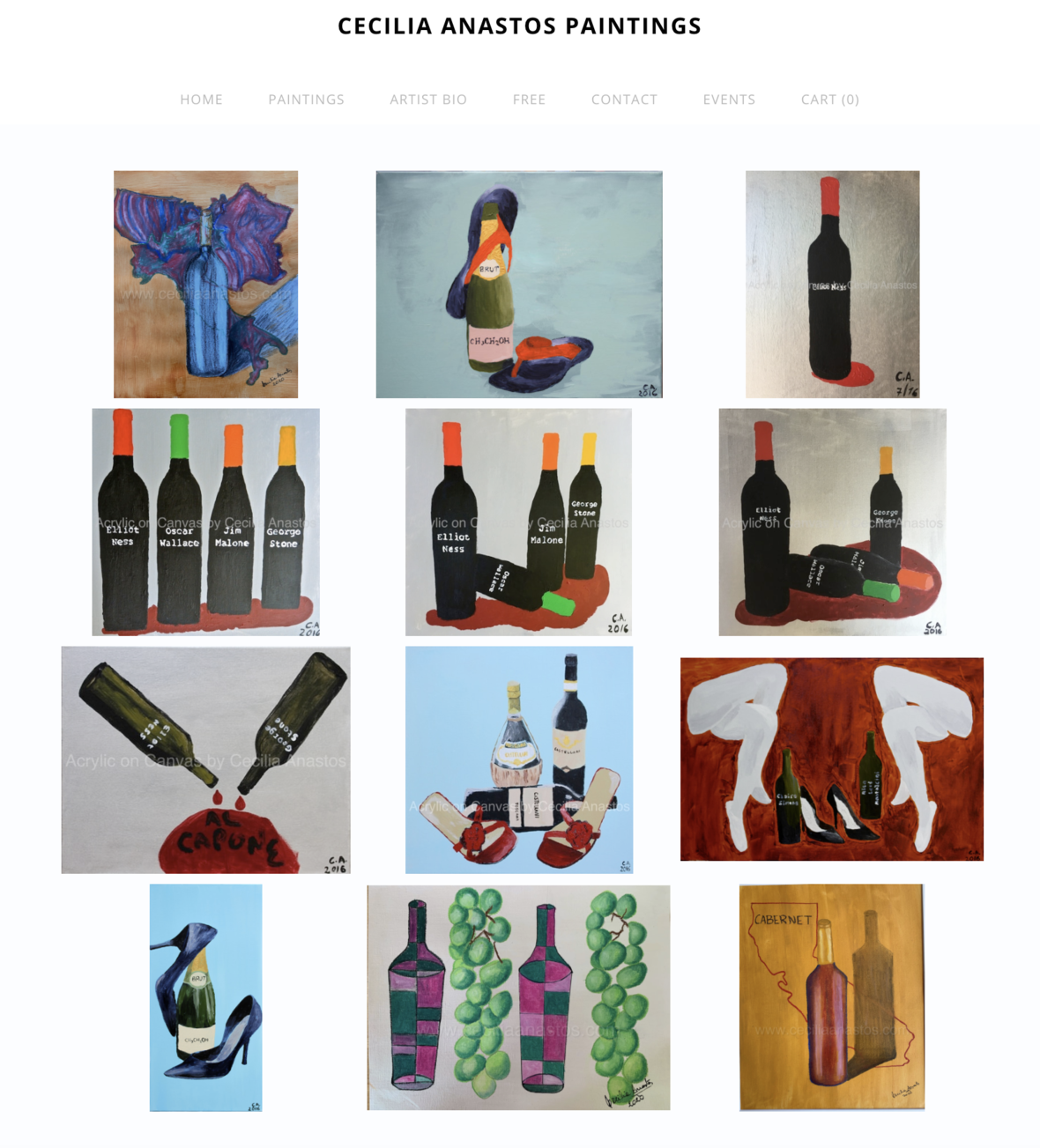From Cecilia Anastos Paintings:
Every year in October, we celebrate wine culture in the Northern Hemisphere. Wine-making is an art and in celebration of that art, artist Cecilia Anastos presents her collection of wine bottle paintings along with a special offer to readers of Shall We Wine.

The full wine bottle paintings collection is available here.

Ms. Anastos expresses her art with acrylic paintings and oil pastels on gallery wrapped canvas or acid free paper. She adds her Italian sense of humor to her artwork - some of the bottles are labeled with the names of The Untouchable men from the Prohibition era, others have the ethanol formula on the label. The artist used wine to create the painting Bacchus (2016).

“If Bacchus ever had a color he could claim for his own, it should surely be the shade of tannin on drunken lips, of John Keat's 'purple-stained mouth', or perhaps even of Homer's dangerously wine-dark sea.” ― Victoria Finlay

Ms. Anastos’ wine bottle collection of paintings is contemporary, figurative and Bohemian style. Most of the paintings in the wine bottle collection are ready to hang, and she offers free shipping in the US.

When asked to select two of her favorite works, she pointed to the Untouchables series, and the Flip Flop Brut. At the end of the movie titled The Untouchables, only Elliott Ness and George Stone remained standing. The painting titled Untouchables – Wallace and Malone Have Fallen (2016) represents that. In her Flip Flop Brut (2016), the label has the ethanol formula and she wanted to express the dichotomy of the elegance usually accompanied by a champagne bottle with the casual attire rendered by the flip flops.

Passion for creating unique paintings drives her inspiration. She is a self-taught artist. She learned long ago to trust the teacher within and to go to her inner world to fetch ideas for paintings.
You can subscribe to the artist’s monthly newsletter at www.ceciliaanastos.com.

Follow the artist on Instagram @ceciliaanastosart; Twitter @AnastosCecilia and LinkedIn @ceciliaanastosart. She can be reached at cecilia@ceciliaanastos.com.

 table div table+table+table+table+table+table+table+table+table+table+table+table+table+table+table+table+table+table+table+table+table+table+table+table+table+table+table+table+table+table+table+table+table+table+table+table+table+table+table div table{width:100%;padding:0}table div table+table+table+table+table+table+table+table+table+table+table+table+table+table+table+table+table+table+table+table+table+table+table+table+table+table+table+table+table+table+table+table+table+table+table+table+table+table+table div table img{width:96.23%;padding:0;float:none}table div table+table+table+table+table+table+table+table+table+table+table+table+table+table+table+table+table+table+table+table+table+table+table+table+table+table+table+table+table+table+table+table+table+table+table+table+table+table+table div table td{width:100%;padding:0 1.88% 18px}/* styles */SHALL WE WINE: THE BLOGWe have a blog! Here you will find things like our Wine of the Week and other special wine & spirits topics! Stay in the know and read up on some of the cool things we've been sharing.

 table div table+table+table+table+table+table+table+table+table+table+table+table+table+table+table+table+table+table+table+table+table+table+table+table+table+table+table+table+table+table+table+table+table+table+table+table+table+table+table+table+table+table div table{width:100%;padding:0}table div table+table+table+table+table+table+table+table+table+table+table+table+table+table+table+table+table+table+table+table+table+table+table+table+table+table+table+table+table+table+table+table+table+table+table+table+table+table+table+table+table+table div table img{width:96.23%;padding:0;float:none}table div table+table+table+table+table+table+table+table+table+table+table+table+table+table+table+table+table+table+table+table+table+table+table+table+table+table+table+table+table+table+table+table+table+table+table+table+table+table+table+table+table+table div table td{width:100%;padding:0 1.88% 18px}/* styles */table div table+table+table+table+table+table+table+table+table+table+table+table+table+table+table+table+table+table+table+table+table+table+table+table+table+table+table+table+table+table+table+table+table+table+table+table+table+table+table+table+table+table+table+table+table div table{width:100%;padding:0}table div table+table+table+table+table+table+table+table+table+table+table+table+table+table+table+table+table+table+table+table+table+table+table+table+table+table+table+table+table+table+table+table+table+table+table+table+table+table+table+table+table+table+table+table+table div table img{width:96.23%;padding:0;float:none}table div table+table+table+table+table+table+table+table+table+table+table+table+table+table+table+table+table+table+table+table+table+table+table+table+table+table+table+table+table+table+table+table+table+table+table+table+table+table+table+table+table+table+table+table+table div table td{width:100%;padding:0 1.88% 18px}/* styles */SWW CONCIERGE SERVICE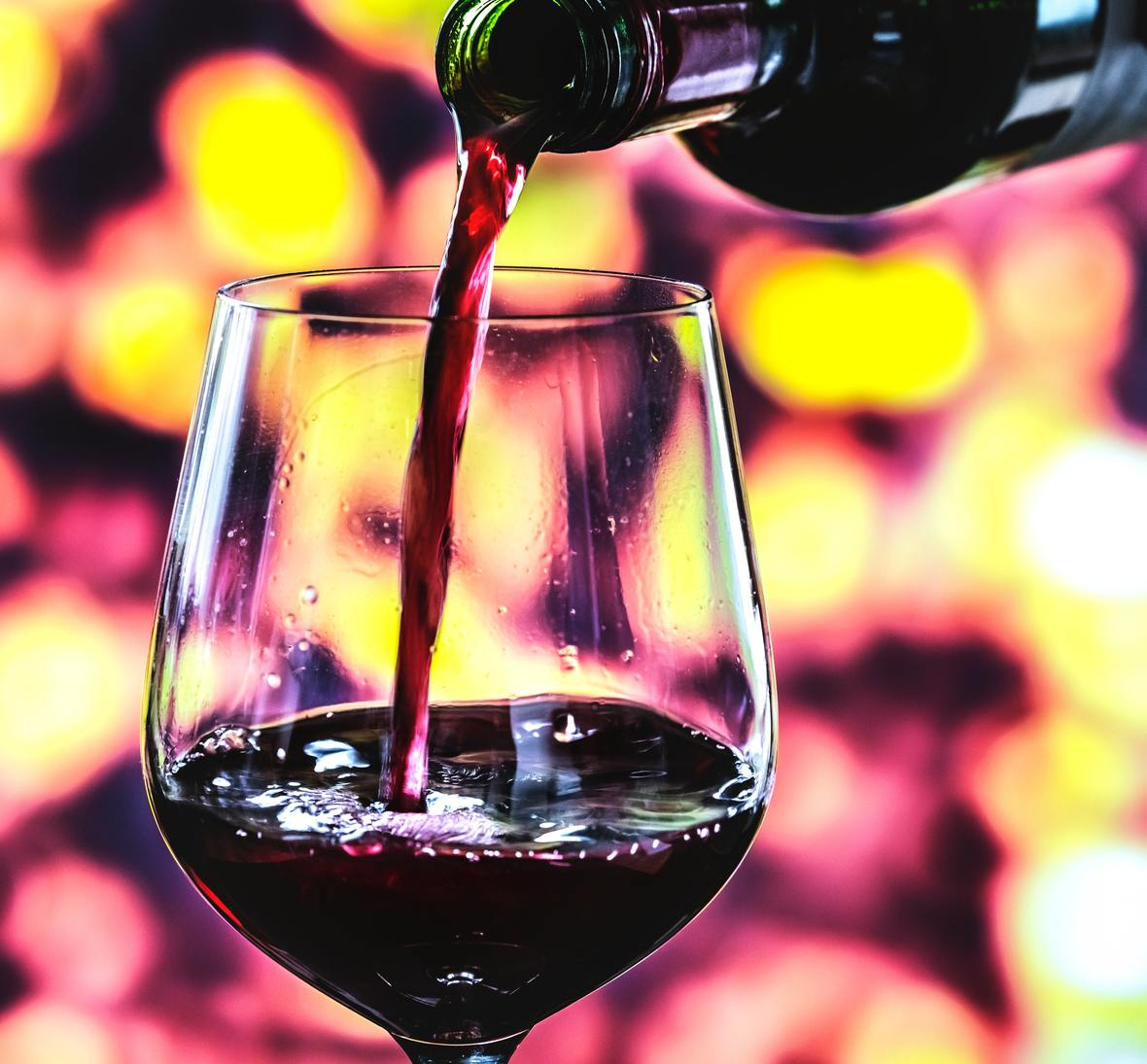Want a custom, tailor-made wine experience?

Sit down (virtually) with Shall We Wine for an exclusive Consultation and Personal Wine Profile! This small group event begins with a wine survey to determine your wine preferences. At the virtual event, we will discuss your wine style. Within a week we will generate a personalized list of 12 wines for you to explore.

 table div table+table+table+table+table+table+table+table+table+table+table+table+table+table+table+table+table+table+table+table+table+table+table+table+table+table+table+table+table+table+table+table+table+table+table+table+table+table+table+table+table+table+table+table+table+table+table+table+table div table{width:100%;padding:0}table div table+table+table+table+table+table+table+table+table+table+table+table+table+table+table+table+table+table+table+table+table+table+table+table+table+table+table+table+table+table+table+table+table+table+table+table+table+table+table+table+table+table+table+table+table+table+table+table+table div table img{width:96.23%;padding:0;float:none}table div table+table+table+table+table+table+table+table+table+table+table+table+table+table+table+table+table+table+table+table+table+table+table+table+table+table+table+table+table+table+table+table+table+table+table+table+table+table+table+table+table+table+table+table+table+table+table+table+table div table td{width:100%;padding:0 1.88% 18px}/* styles */THANKS AND TOGETHERNESS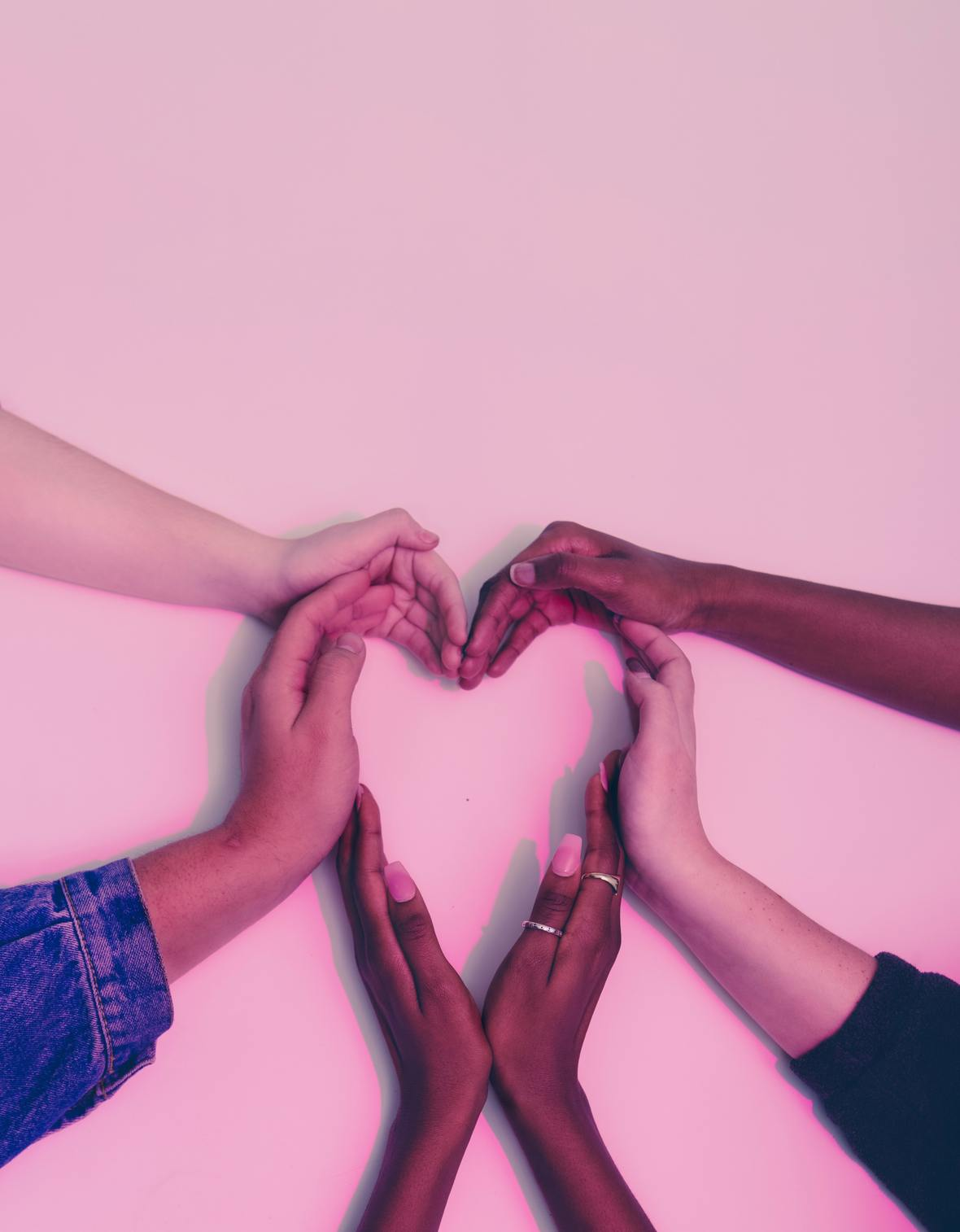In an effort to maintain social distancing measures, Shall We Wine will continue to devote our time to connecting with each and every one of our friends and supporters like you virtually. We're constantly sharing fun content to help brighten up your weeks!

We appreciate your patience and cooperation as we work through these times together. Thank you for your continued support in making Shall We Wine your go-to wine & lifestyle resource!/* styles */ Learn, Drink, Repeat! The Shall We Wine Team
 Like   Tweet   Pin   +1   in